Is it possible for a neural network to learn how to talk like humans? Recent advances in recurrent neural networks allow us to model a language, by predicting what words will come next given a context.

I decided to try and simulate the typical Twitch viewer. Type a few words into the box below and press enter to see what the model predicts a Twitch viewer would say.

In this post, I'll go over a brief explanation of recurrent neural networks, and put a focus on how the data was collected and formatted, as well as some analysis on how well the model did.

Now, you might be asking, why Twitch?

Oftentimes, the hardest part of solving a machine learning problem is collecting the data. Well, Twitch is always running, so I could write a simple node.js scraper to download the messages as they came up.

Second, Twitch chat often contains a lot of memes and copypasta, and I wanted to see if the network could learn to complete the copypasta if given the beginning.

Finally, messages on Twitch aren't as dependent on the previous messages since the chat is moving so fast, so I could treat each message independently.

#### How does it work?

The model this time is a recurrent neural network. In short, it's a neural network that repeats over timesteps, and passes data into itself every iteration.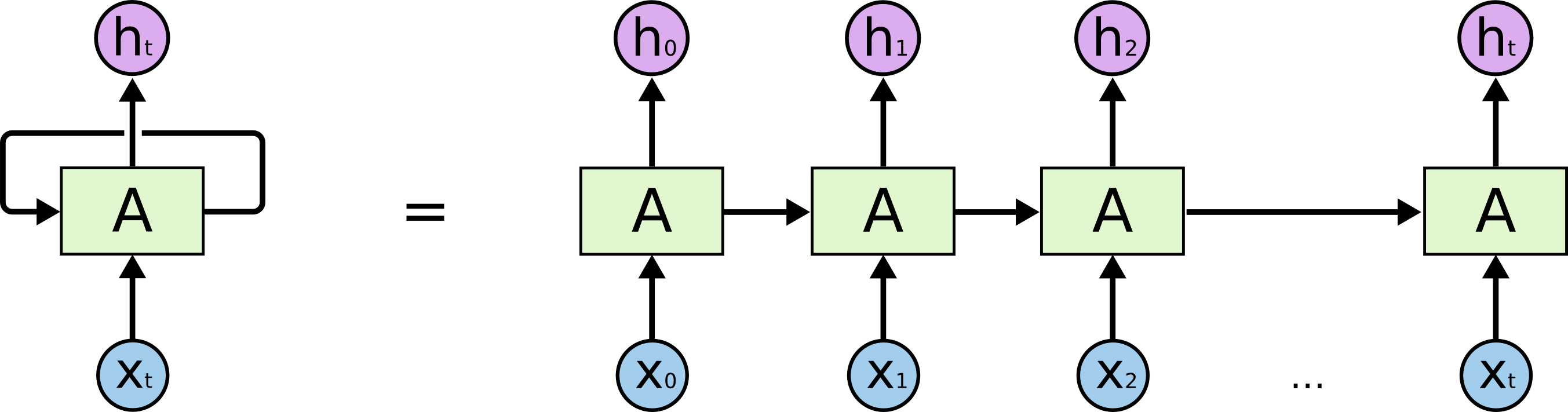image source

Here, X is a vector that contains the words in the beginning of a sentence. The words are encoded as IDs, so passing "league of" would be seen as [545, 23], if "league" is the 545th word, etc.

We want to predict the next word, so passing in "league of" would give an output of "legends". The output of each layer is a vector of [vocab size x 1], with each value being the probability of that specific word coming next in the sentence. We can then either take a sample of these probabilities to choose the next word, or just take the maximum.

The green box is a single hidden layer that takes in two inputs: the current word, and the value of the previous hidden layer. They all share the same weights, so we can extend the recurrent network as long as we need to. In practice, we use an LSTM instead of a fully-connected layer, but their purpose is the same.

To train the network, we use a cross-entropy loss on the prediction of each word in the message. This backpropogates through to the beginning from each word, allowing the network to train multiple times from one sentence.

This setup is pretty typical, so I won't go into much detail. Check out the source code or this post by Andrej Karpathy for more explanations.

#### Working with Data

Here's what I would consider the trickiest part of the setup. We know we want to use Twitch chat as a source of data, but how do we actually do that in practice?

First comes the actual collection. I used a scraper in node.js to read the IRC channels that Twitch operates on, and save every message to a text file.

``````// tmi is an npm module for reading Twitch chat
var client = new tmi.client(options);
var totalstring = "";
client.on("chat", function (channel, userstate, message, self) {
//remove non-alphanumeric or space characters
message = message.replace(/[^\w\s]/gi, '')
//marker for end of sentence
message = message + "<eos>"
totalstring += message
fs.writeFile("database.txt", totalstring, function(err) {});
}
``````

The important thing to take away here is to always clean your data. On the first iteration I didn't remove weird characters, and some ASCII art totally messed up the dataset.

Next, we need to convert the sentence to arrays of word IDs. The first step is to decide on which IDs to should map to which words.

``````data = totalstring.toLowerCase();
var sentences = data.split("<eos>");
for(var s = 0; s < sentences.length; s++)
{
sentences[s].replace(/[^0-9a-zA-Z ]/g, '')
var words = sentences[s].split(" ");
for(var w = 0; w < words.length; w++)
{
if(topwords[words[w]] == null)
{
topwords[words[w]] = 1;
}
else
{
topwords[words[w]] = topwords[words[w]] + 1;
}
}
}
var len = Object.keys(topwords).length;
var thewords = Object.keys(topwords);
for(var w = 0; w < len; w++)
{
if(topwords[thewords[w]] < 5)
{
delete topwords[thewords[w]];
}
else
{
topwords[thewords[w]] = w+1;
}
}
jsonfile.writeFile("words.json", topwords, function (err) {});
``````

Here, we're taking counts of how many times each word appears. If it appeared less than 5 times, we discard it. Later on, we'll add a special word that will represent all rare words in sentences.

If the word occurs enough times, we keep it and assign it an ID number that counts up from 1. The number 0 is reserved for when there is no word.

Now, we'll switch to python to construct a numpy matrix containing the word IDs for each message.

``````with open('database.txt') as data_file:

with open('words.json') as data_file:

count = 0
split_sentences = sentences.split("<eos>")
# 152401 sentences
# 33233 words
nparray = np.zeros((152401,20))

for s in xrange(len(split_sentences)):
sentence = split_sentences[s].lower()
regex = re.compile('[^0-9a-zA-Z ]')
realsent = regex.sub('', sentence)
words = sentence.split(" ")
if len(words) >= 2:
count = count + 1
for w in xrange(min(len(words),20)):
word = words[w]
if word in wordsdata:
nparray[count][w] = wordsdata[word]
else:
# its a rare word
nparray[count][w] = 33234

nparray = nparray[:count,:]
np.random.shuffle(nparray)
np.save("data.npy",nparray)
``````

Any sentences that only have two or less words are discarded. If there's a rare word, we replace it with a special id 33234, which is [vocabsize + 1]. We set the maximum message length to 20. If the message ends before that, which most do, the rest of the vector is filled with 0's.

It's important to shuffle the rows in the end, in case there is any correlation between messages scraped at similar times (which there often is). This might cause the neural network to jump back and forth during training.

After all of that, we're finally done with data preparation and sanitization! I'm sure there are many improvements that could be made in my methods, but it worked well enough.

Data handling is a part of the process that normally gets left out when talking about machine learning, but it's an important part of the process as well.

#### How well did it do?

Well, did the network work? You can try out whatever phrases you want in the field at the top of the page.

Since there's no concrete way to measure how well the model performs, here are some examples that might shine some light.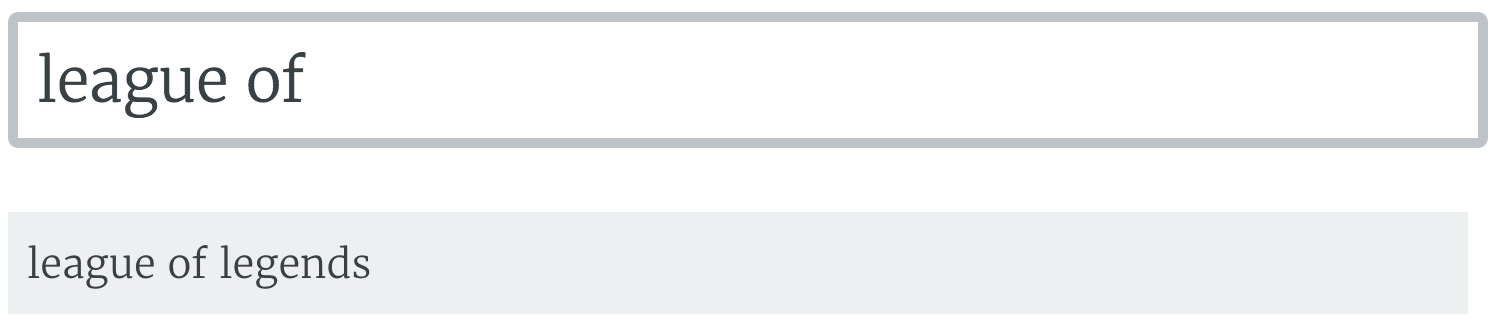It was able to learn the names of a few popular Twitch games, since they were said a lot.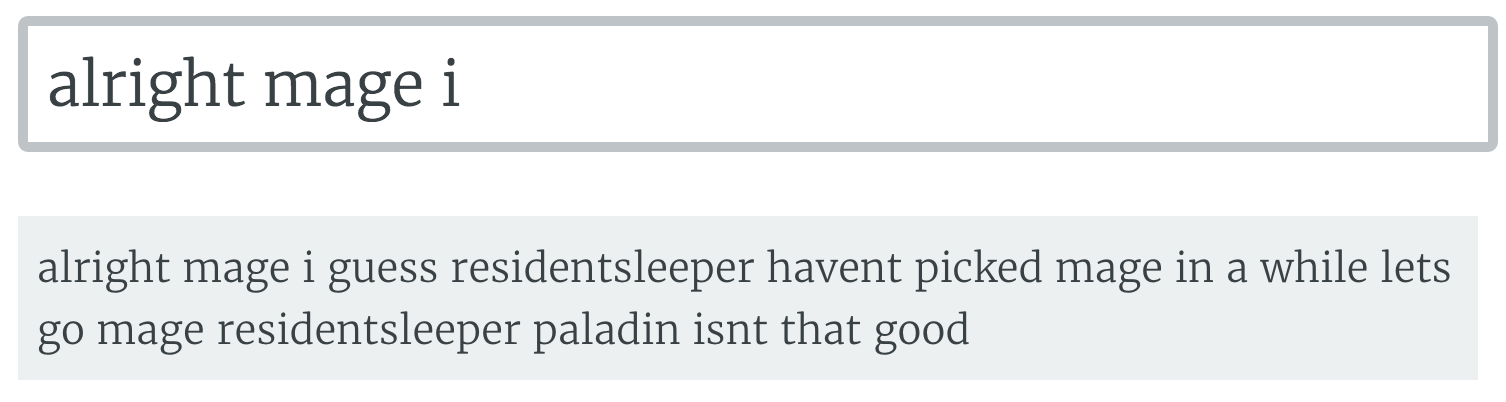A copypasta from Kripp's stream. Even though I started the message from the middle of the copypasta, the model was able to recognize it and finish the job.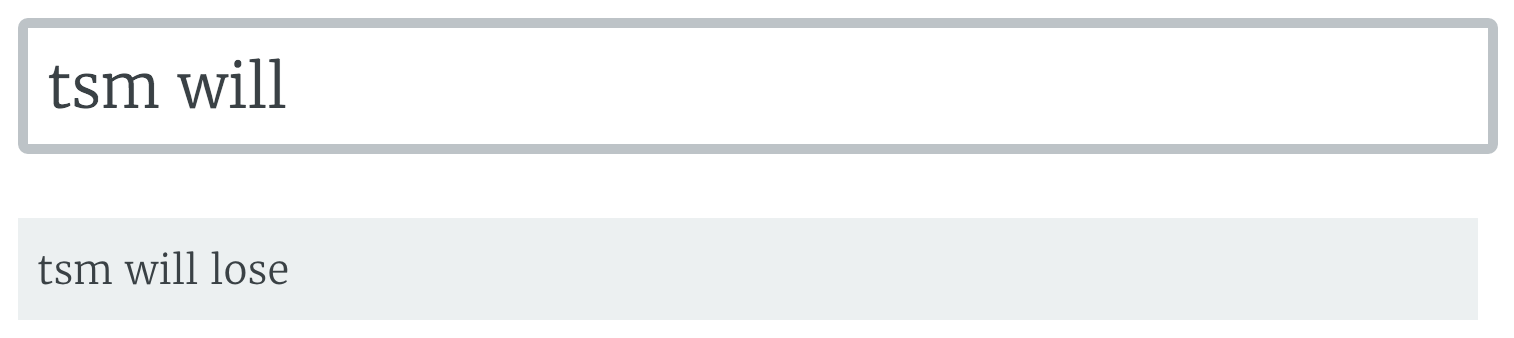Got some nice banter here.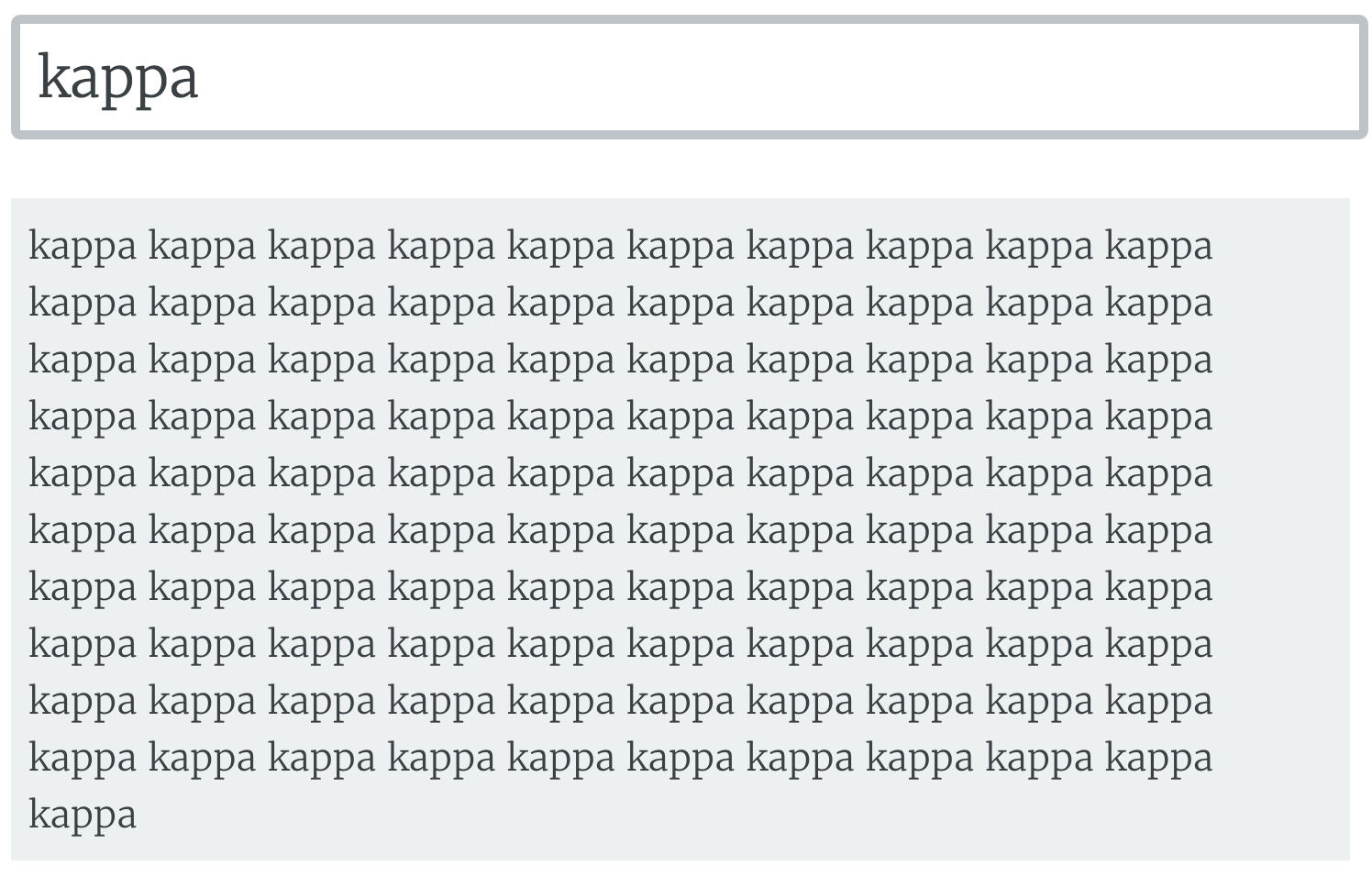Putting in an emote usually just gives an endless loop of emotes. It's imitating chat spamming kappa all the time.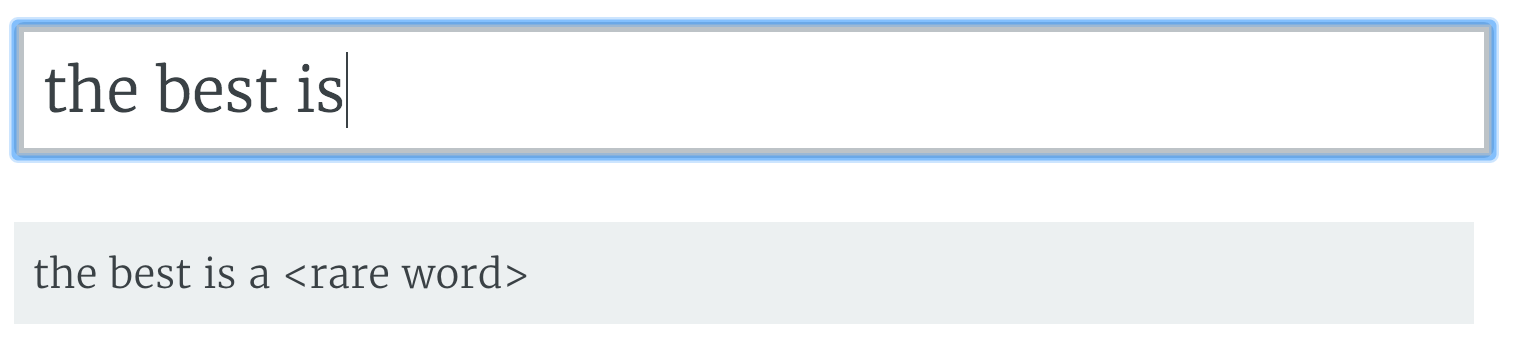If you put in some generic phrase, the network usually responds with `<rare word>`. This is probably due to the total number of rare words outnumbering any single word in the dataset. It did learn to put an "a" before the word, though.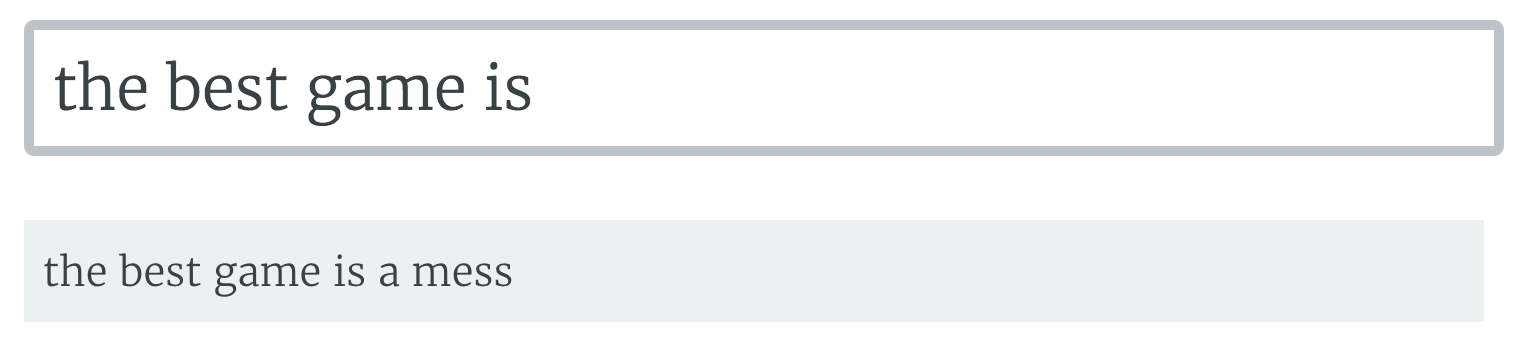Adding better context gives better results, along with some deep thoughts about game design.

Overall, the model did perform pretty well. A nice way it could be tested is to use some form of a Turing test where we make a Twitch bot say phrases from the neural network, and see if anyone catches on.

The code for this project is available on my Github, along with the 5MB dataset I scraped.

Thanks Michael and Kevin for helping build the first trial of this